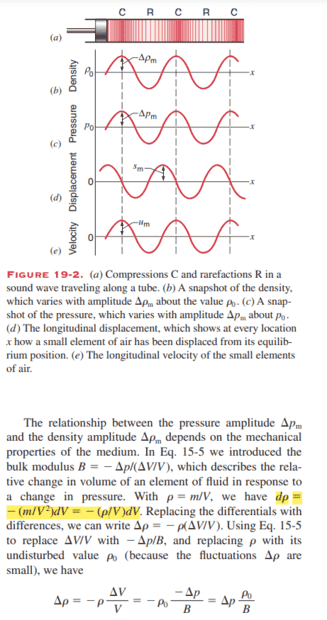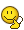# Solving a Sound Wave Equation in Physics 1: Halliday, Resnick, and Krane

• Afo
In summary, the conversation is about understanding an equation from page 428 on sound waves in a Physics textbook. The equation involves density and differentiation, and the author is trying to understand how it was derived. The conversation also includes a discussion on derivatives and differentials, with the final conclusion being that the equation can be understood by using the fact that density is a function of volume and the derivative of this function is equal to -m/V^2.f

#### Afo

Homework Statement:: This is from 5 ed, Physics 1Halliday, Resnick, and Krane. page 428 about sound waves

I have highlighted the equation that I don't understand. How did the author get it? I understand how they get from the middle side to the RHS of the equation, but I don't understand how they get from the first equation to the middle equation.

I don't have any problem with anything before.
Relevant Equations:: Density: Rho = Mass / VolumeDifferentiation.

•Afo and vanhees71
Differentiation.
Is it like this?

dm/dv^2 = - m /(V^2)
so
dp = dm/dv = dv * (-m/(V^2))

No. Use ##\Delta y/\Delta x \simeq dy/dx##

•Afo
No. Use ##\Delta y/\Delta x \simeq dy/dx##

Like this?
##d\rho = \frac{\Delta \rho}{\Delta V}dV##
but ##\frac{\Delta \rho}{\Delta V} = -\frac{m}{V^2}##

•vanhees71
Is it like this?

dm/dv^2 = - m /(V^2)
so
dp = dm/dv = dv * (-m/(V^2))
Okay, I know why this is false.
It doesn't work since ##\frac{a}{b} = \frac{c}{d}## doesn't imply ## \frac{a}{b^2} = \frac{c}{d^2}.## If that was true, that would mean ##|dV| = |V|## which is obviously false.

Do you know how a derivative works?

•Afo
Do you know how a derivative works?
I am still in high school so this is what I know:

1. We can view ##\frac{d}{dx}## as an operator so ##\frac{d}{dx}f(x) = \frac{df}{dx}## is the derivative of ##f## w.r.t. ##x##.

Another way is to view it in terms of differentials:
2. ##dx## and ##dy## are called differentials. The differential ##dx## is an independent variable. The differential ##dy## is defined to be ##dy = f'(x) dx##

Both ways are rigorous.

So, let ##x = V##, ##y = \rho(V)## and use 2 to relate ##d\rho## to ##dV##.

•Afo
So, let ##x = V##, ##y = \rho(V)## and use 2 to relate ##d\rho## to ##dV##.Ok, I got it!

##\rho(V) = \frac{m}{V}## is a function of volume and ##m## is a constant.

Thus ##\frac{d}{dV} \rho(V) = \frac{d}{dV} \frac{m}{V} = m\frac{d}{dV} \frac{1}{V} = -\frac{m}{V^2}##

•vanhees71, Orodruin and TSny